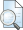## PENERAPAN MATRIKS HOUSE HOLDER PADA SISTEM PERSAMAAN LINEAR TAK KONSISTEN

Ari Setiawan, Adytia (2010) PENERAPAN MATRIKS HOUSE HOLDER PADA SISTEM PERSAMAAN LINEAR TAK KONSISTEN. Other thesis, University of Muhammadiyah Malang.Preview
Text

## Abstract

System of linear equations can be written in matrix form where is a coefficient matrix order , is a column matrix which its element represent variable with order , and is a column matrix which its element represent constanta with order . Column matrix and on the system can be regarded as a column vector. System of linear equations can be divided into two systems that is consistent system and inconsistent systems. Consistent system is a system that has a solution and inconsistent systems is a system which does not have a solution. At the writing of this skripsi focused on the inconsistent systems was to amount of equation more than its amount of variable. In inconsistent system can not find a vector such that equal to . But can be searched a vector such that approaches . That solution is called the least squares solution. Searching squares solutions from equivalent to finding solutions of with as Householder matrix order which defined as with as column vector non zero in and as identity matrix. Keyword : Householder matrix, least squares solutions.

Item Type: Thesis (Other) L Education > L Education (General) Faculty of Teacher Training and Education > Department of Mathematics Education (84202) Rayi Tegar Pamungkas 04 Apr 2012 02:06 04 Apr 2012 02:06 http://eprints.umm.ac.id/id/eprint/1782View Item